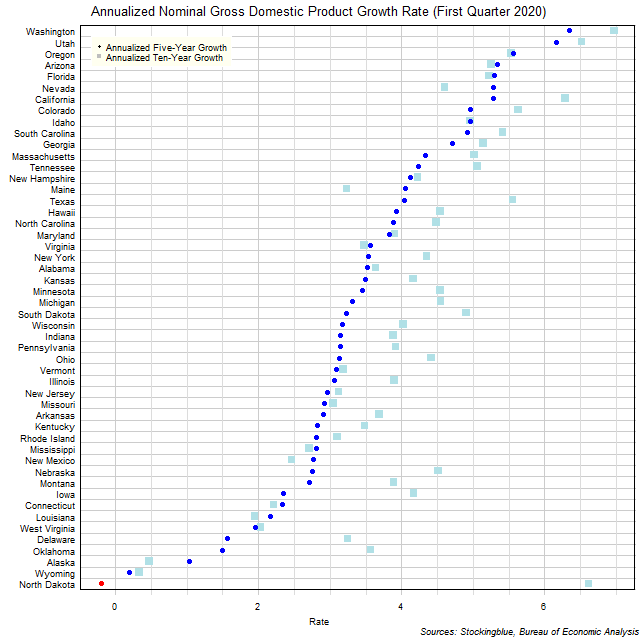# Long-Term US GDP Growth Rate by State, First Quarter 2020

Sep 16, 2020The chart above shows the annualized nominal gross domestic product (GDP) growth rate in each US state over the past five years as of the first quarter of 2020 and the growth rate over the past ten years.  Only one state experienced negative growth in either time period.

## Findings

• The difference between the state with the largest annualized five-year growth rate, Washington, and the state with the smallest, North Dakota, is 6.55 percentage points.
• The difference between the state with the largest annualized ten-year growth rate, Washington, and the state with the smallest, Wyoming, is 6.64 percentage points.
• The median annualized five-year growth rate in the 50 US states is 3.27% and the mean 3.45%.
• The median annualized ten-year growth rate in the 50 US states is 4.17% and the mean 4.10%.
• Eleven states' economies grew faster over the past five years than they did over the past ten years.  No state had the same annualized growth rate over both periods.  The remaining 39 states saw their economies grow slower over the past five years than they did over the past ten years.

## Caveats

• Data is from the first quarters of 2010, 2015, and 2020.
• The data is seasonally adjusted in current dollars.
• Growth rates may differ from those provided by the Bureau of Economic Analysis as the BEA's growth rates are based on chained dollars in conjunction with the chain index or the quality index for real GDP.
• All figures are rounded to the nearest hundredth.

## Details

North Dakota had the worst performance over the past five years with an annualized growth rate of -0.20%.  Washington had the best performance with an annualized gain of 6.35%.

Over the past ten years, Wyoming had the worst performance with an annualized growth rate of 0.34% while Washington had the best performance with an annualized growth rate of 6.97%.

North Dakota had the worst change in its growth rate between its annualized ten-year growth and its annualized five-year growth slowing its rate by 6.82 percentage points.  Maine had the best change in its growth rate between its annualized ten-year growth and its annualized five-year growth ramping up its rate by 0.82 percentage points.

Maine went from having the 39th largest annualized growth rate over the past ten years to the 15th largest annualized growth rate over the past five years.  Conversely, North Dakota went from having the 2nd highest annualized growth rate over the past ten years to the 50th highest annualized growth rate over the past five years.  Only two states had annualized growth rates in the top five both over the past ten years and over the past five years: Washington (highest annualized growth over ten years, highest annualized growth over five years) and Utah (3rd highest ten-year, 2nd highest five-year).  Two states have ranked in the bottom five over both periods: Alaska (49th ten-year, 48th five-year) and Wyoming (50th ten-year, 49th five-year).

## Sources

US Bureau of Economic Analysis.  2020.  "GDP by State."  Accessed August 19, 2020.  https://www.bea.gov/data/gdp/gdp-state.

Filed under: Charts and Graphs